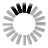The Boolean logic function of interest can be specified in different manners:
1. providing a well-formed formula of propositional calculus
2. providing a binary number.

How to write a well-formed formula of propositional calculus:
• Any type of letters can be used as variable.
• ! should be used as negation (negation): if A is a well-formed formula, !A is also well-formed.
• + as sum (logical disjunction): if A and B are well-formed formulas, so is A+B.
• . as product (logical conjunction): if A and B are well-formed formulas, so is A.B.
• ( and ) can be used to prioritize a sub-formula: if A is a well-formed formula, (A) is also well-formed.
The priority of the operators is !, ., +.

The binary number (a list of 0 and 1 of length of 2n with n the number of inputs) corresponds to the list of outputs of the different input states (it represents the last column of a truth table of a well-formed formula).

For example, the two-input logic function XOR (exclusive or) can be writen with the well-formed formula !a.b+!b.a or with the binary number 0110.
It is also possible to specify multiple functions simultaneously by writing the corresponding well-formed formulas in different lines.